1604195640

# Kaggle House Prices Prediction with Linear Regression and Gradient Boosting

**My **Kaggle Notebook Link is here

### As I intended this Notebook to be published as a blog on Linear Regression, Gradient Descent function and some EDA, so in the first 50% to 60% of this notebook I have mainly discussed the theory around those topics from mainly a beginner perspective.

``````import numpy as np
import pandas as pd
import matplotlib.pyplot as plt
import seaborn as sns
from sklearn.pipeline import Pipeline
from sklearn.preprocessing import LabelEncoder, OneHotEncoder
from sklearn.compose import ColumnTransformer
from sklearn.linear_model import LinearRegression
from sklearn.linear_model import Ridge
from sklearn.linear_model import Lasso
from sklearn.ensemble import RandomForestRegressor
from sklearn.impute import SimpleImputer
from sklearn.preprocessing import StandardScaler
from sklearn.model_selection import train_test_split
from sklearn import metrics
from sklearn.metrics import mean_squared_log_error
from sklearn.metrics import mean_squared_error
from xgboost import XGBRegressor
import math
``````

The evaluation criteria for this Kaggle Competition is RMSLE — “Submissions are evaluated on Root-Mean-Squared-Error (RMSE) between the logarithm of the predicted value and the logarithm of the observed sales price. (Taking logs means that errors in predicting expensive houses and cheap houses will affect the result equally.)”

The Root Mean Squared Log Error (RMSLE) can be defined using a slight modification on sklearn’s mean_squared_log_error function, which itself a modification on the familiar Mean Squared Error (MSE) metric.

``````def root_mean_squared_log_error(y_validations, y_predicted):
if len(y_predicted) != len(y_validations):
return 'error: mismatch in number of data points between x and y'
y_predict_modified = [math.log(i) for i in y_predicted]
y_validations_modified = [math.log(i) for i in y_validations]

return mean_squared_error(y_validations_modified, y_predict_modified, squared=False)
``````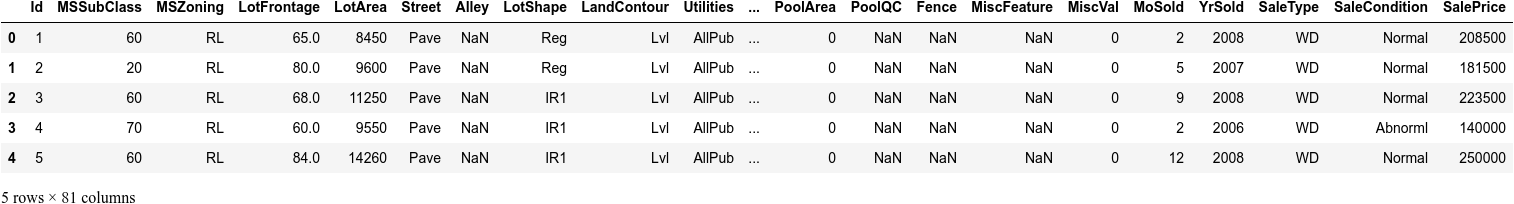``````df_test.head()
``````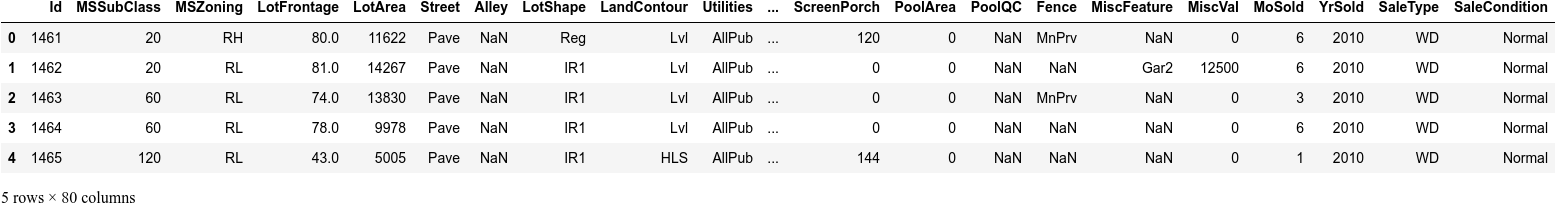First, I am getting a basic description of the data, to look quickly at the overall data to get some simple, easy-to-understand information. This is just to get a basic feel for the data. Using describe() function to get various summary statistics that exclude NaN values.

``````df_train.describe()
``````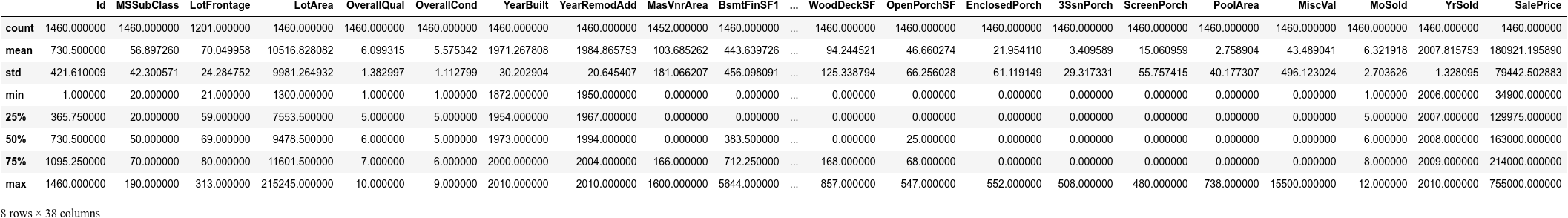#kaggle #data-science #kaggle-competition #python #machine-learning

## Buddha Community1604195640

## Kaggle House Prices Prediction with Linear Regression and Gradient Boosting

**My **Kaggle Notebook Link is here

### As I intended this Notebook to be published as a blog on Linear Regression, Gradient Descent function and some EDA, so in the first 50% to 60% of this notebook I have mainly discussed the theory around those topics from mainly a beginner perspective.

``````import numpy as np
import pandas as pd
import matplotlib.pyplot as plt
import seaborn as sns
from sklearn.pipeline import Pipeline
from sklearn.preprocessing import LabelEncoder, OneHotEncoder
from sklearn.compose import ColumnTransformer
from sklearn.linear_model import LinearRegression
from sklearn.linear_model import Ridge
from sklearn.linear_model import Lasso
from sklearn.ensemble import RandomForestRegressor
from sklearn.impute import SimpleImputer
from sklearn.preprocessing import StandardScaler
from sklearn.model_selection import train_test_split
from sklearn import metrics
from sklearn.metrics import mean_squared_log_error
from sklearn.metrics import mean_squared_error
from xgboost import XGBRegressor
import math
``````

The evaluation criteria for this Kaggle Competition is RMSLE — “Submissions are evaluated on Root-Mean-Squared-Error (RMSE) between the logarithm of the predicted value and the logarithm of the observed sales price. (Taking logs means that errors in predicting expensive houses and cheap houses will affect the result equally.)”

The Root Mean Squared Log Error (RMSLE) can be defined using a slight modification on sklearn’s mean_squared_log_error function, which itself a modification on the familiar Mean Squared Error (MSE) metric.

``````def root_mean_squared_log_error(y_validations, y_predicted):
if len(y_predicted) != len(y_validations):
return 'error: mismatch in number of data points between x and y'
y_predict_modified = [math.log(i) for i in y_predicted]
y_validations_modified = [math.log(i) for i in y_validations]

return mean_squared_error(y_validations_modified, y_predict_modified, squared=False)
````````````df_test.head()
``````First, I am getting a basic description of the data, to look quickly at the overall data to get some simple, easy-to-understand information. This is just to get a basic feel for the data. Using describe() function to get various summary statistics that exclude NaN values.

``````df_train.describe()
``````#kaggle #data-science #kaggle-competition #python #machine-learning1594271340

## A Deep Dive into Linear Regression

Let’s begin our journey with the truth — machines never learn. What a typical machine learning algorithm does is find a mathematical equation that, when applied to a given set of training data, produces a prediction that is very close to the actual output.

Why is this not learning? Because if you change the training data or environment even slightly, the algorithm will go haywire! Not how learning works in humans. If you learned to play a video game by looking straight at the screen, you would still be a good player if the screen is slightly tilted by someone, which would not be the case in ML algorithms.

However, most of the algorithms are so complex and intimidating that it gives our mere human intelligence the feel of actual learning, effectively hiding the underlying math within. There goes a dictum that if you can implement the algorithm, you know the algorithm. This saying is lost in the dense jungle of libraries and inbuilt modules which programming languages provide, reducing us to regular programmers calling an API and strengthening further this notion of a black box. Our quest will be to unravel the mysteries of this so-called ‘black box’ which magically produces accurate predictions, detects objects, diagnoses diseases and claims to surpass human intelligence one day.

We will start with one of the not-so-complex and easy to visualize algorithm in the ML paradigm — Linear Regression. The article is divided into the following sections:

1. Need for Linear Regression

2. Visualizing Linear Regression

3. Deriving the formula for weight matrix W

4. Using the formula and performing linear regression on a real world data set

Note: Knowledge on Linear Algebra, a little bit of Calculus and Matrices are a prerequisite to understanding this article

Also, a basic understanding of python, NumPy, and Matplotlib are a must.

## 1) Need for Linear regression

Regression means predicting a real valued number from a given set of input variables. Eg. Predicting temperature based on month of the year, humidity, altitude above sea level, etc. Linear Regression would therefore mean predicting a real valued number that follows a linear trend. Linear regression is the first line of attack to discover correlations in our data.

Now, the first thing that comes to our mind when we hear the word linear is, a line.

Yes! In linear regression, we try to fit a line that best generalizes all the data points in the data set. By generalizing, we mean we try to fit a line that passes very close to all the data points.

But how do we ensure that this happens? To understand this, let’s visualize a 1-D Linear Regression. This is also called as Simple Linear Regression

#calculus #machine-learning #linear-regression-math #linear-regression #linear-regression-python #python1595563500

## House Prices Prediction Using Deep Learning

In this tutorial, we’re going to create a model to predict House prices🏡 based on various factors across different markets.

# Problem Statement

The goal of this statistical analysis is to help us understand the relationship between house features and how these variables are used to predict house price.

# Objective

• Predict the house price
• Using two different models in terms of minimizing the difference between predicted and actual rating

Data used: Kaggle-kc_house Dataset

GitHub: you can find my source code here

# Step 1: Exploratory Data Analysis (EDA)

First, Let’s import the data and have a look to see what kind of data we are dealing with:

``````#import required libraries
import pandas as pd
import numpy as np
import seaborn as sns
import matplotlib.pyplot as plt
#import Data
#get some information about our Data-Set
Data.info()
Data.describe().transpose()
``````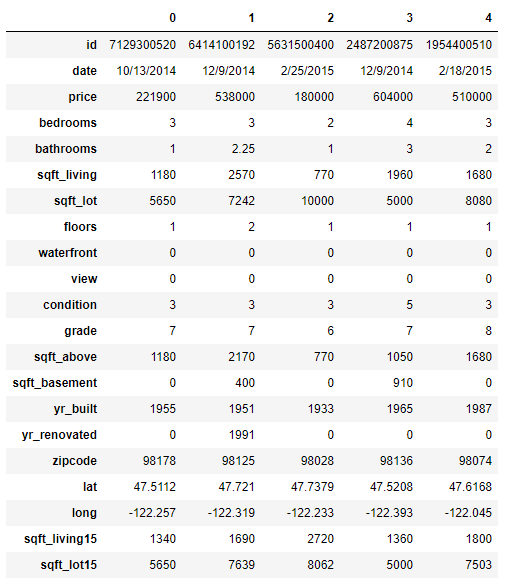5 records of our dataset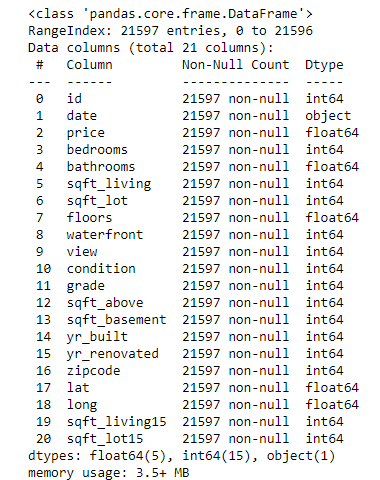Information about the dataset, what kind of data types are your variables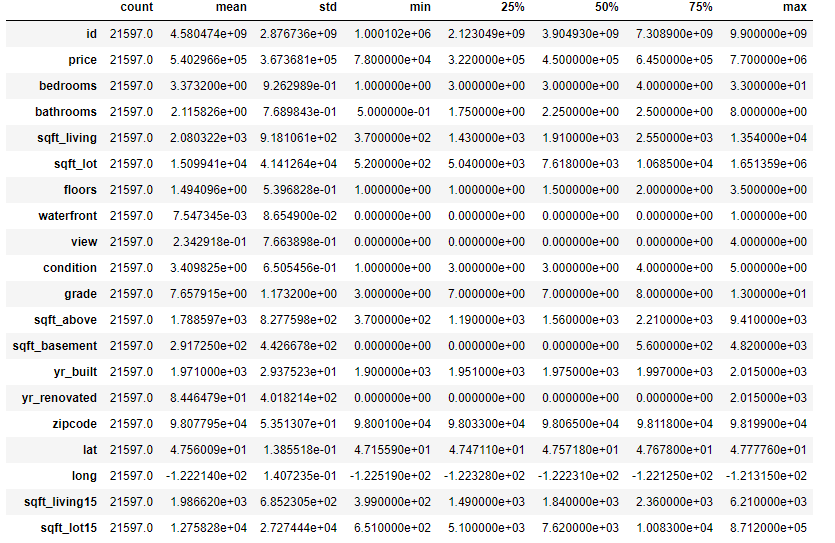Our features are:

✔️**Date:**_ Date house was sold_

✔️**Price:**_ Price is prediction target_

✔️**_Bedrooms: _**Number of Bedrooms/House

✔️**Bathrooms:**_ Number of bathrooms/House_

✔️**Sqft_Living:**_ square footage of the home_

✔️**Sqft_Lot:**_ square footage of the lot_

✔️**Floors:**_ Total floors (levels) in house_

✔️**Waterfront:**_ House which has a view to a waterfront_

✔️**View:**_ Has been viewed_

✔️**Condition:**_ How good the condition is ( Overall )_

✔️**Sqft_Above:**_ square footage of house apart from basement_

✔️**Sqft_Basement:**_ square footage of the basement_

✔️**Yr_Built:**_ Built Year_

✔️**Yr_Renovated:**_ Year when house was renovated_

✔️**Zipcode:**_ Zip_

✔️**Lat:**_ Latitude coordinate_

✔️**_Long: _**Longitude coordinate

✔️**Sqft_Living15:**_ Living room area in 2015(implies — some renovations)_

✔️**Sqft_Lot15:**_ lotSize area in 2015(implies — some renovations)_

Let’s plot couple of features to get a better feel of the data

``````#visualizing house prices
fig = plt.figure(figsize=(10,7))
sns.distplot(Data['price'])
sns.boxplot(Data['price'])
plt.tight_layout()
#visualizing square footage of (home,lot,above and basement)
fig = plt.figure(figsize=(16,5))
sns.scatterplot(Data['sqft_above'], Data['price'])
sns.scatterplot(Data['sqft_lot'],Data['price'])
sns.scatterplot(Data['sqft_living'],Data['price'])
sns.scatterplot(Data['sqft_basement'],Data['price'])
fig = plt.figure(figsize=(15,7))
sns.countplot(Data['bedrooms'])
sns.countplot(Data['floors'])
sns.countplot(Data['bathrooms'])
plt.tight_layout()
``````

With distribution plot of price, we can visualize that most of the prices are between 0 and around 1M with few outliers close to 8 million (fancy houses😉). It would make sense to drop those outliers in our analysis.

#linear-regression #machine-learning #python #house-price-prediction #deep-learning #deep learning1592023980

## 5 Regression algorithms: Explanation & Implementation in Python

Take your current understanding and skills on machine learning algorithms to the next level with this article. What is regression analysis in simple words? How is it applied in practice for real-world problems? And what is the possible snippet of codes in Python you can use for implementation regression algorithms for various objectives? Let’s forget about boring learning stuff and talk about science and the way it works.

#linear-regression-python #linear-regression #multivariate-regression #regression #python-programming1598352300

## Regression: Linear Regression

Machine learning algorithms are not your regular algorithms that we may be used to because they are often described by a combination of some complex statistics and mathematics. Since it is very important to understand the background of any algorithm you want to implement, this could pose a challenge to people with a non-mathematical background as the maths can sap your motivation by slowing you down.In this article, we would be discussing linear and logistic regression and some regression techniques assuming we all have heard or even learnt about the Linear model in Mathematics class at high school. Hopefully, at the end of the article, the concept would be clearer.

**Regression Analysis **is a statistical process for estimating the relationships between the dependent variables (say Y) and one or more independent variables or predictors (X). It explains the changes in the dependent variables with respect to changes in select predictors. Some major uses for regression analysis are in determining the strength of predictors, forecasting an effect, and trend forecasting. It finds the significant relationship between variables and the impact of predictors on dependent variables. In regression, we fit a curve/line (regression/best fit line) to the data points, such that the differences between the distances of data points from the curve/line are minimized.

#regression #machine-learning #beginner #logistic-regression #linear-regression #deep learning